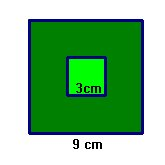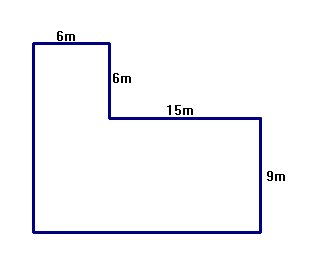WORKSHEET: AREAS 1

Worksheets Descargar PDF
1) What is the area of a triangle with a base of 10 decimetres and a height of 7 decimetres?
Solution
2)What is the area of a parallelogram with bases that are 12 cm and a height of 8 cm?
Solution
3)What is the area of a triangle whose base is one half its height if the height is 14 dm?
Solution
4) What is the length of a rectangle if the area is 35 square metres and the height is 5 metres?
Solution
5) If a parallelogram has an area of 40 square metres and a base of 8 m, what is its height?

Solution
6) What is the area of an equilateral triangle with a base of 5 metres?
Solution
7) Calculate the area of the shaded portions:
 a)Solution
 b)Solution
 c)Solution
8) Calculate the area of the following figure:
 e)Solution